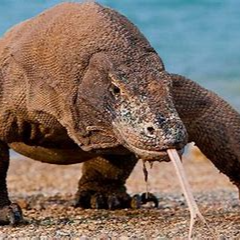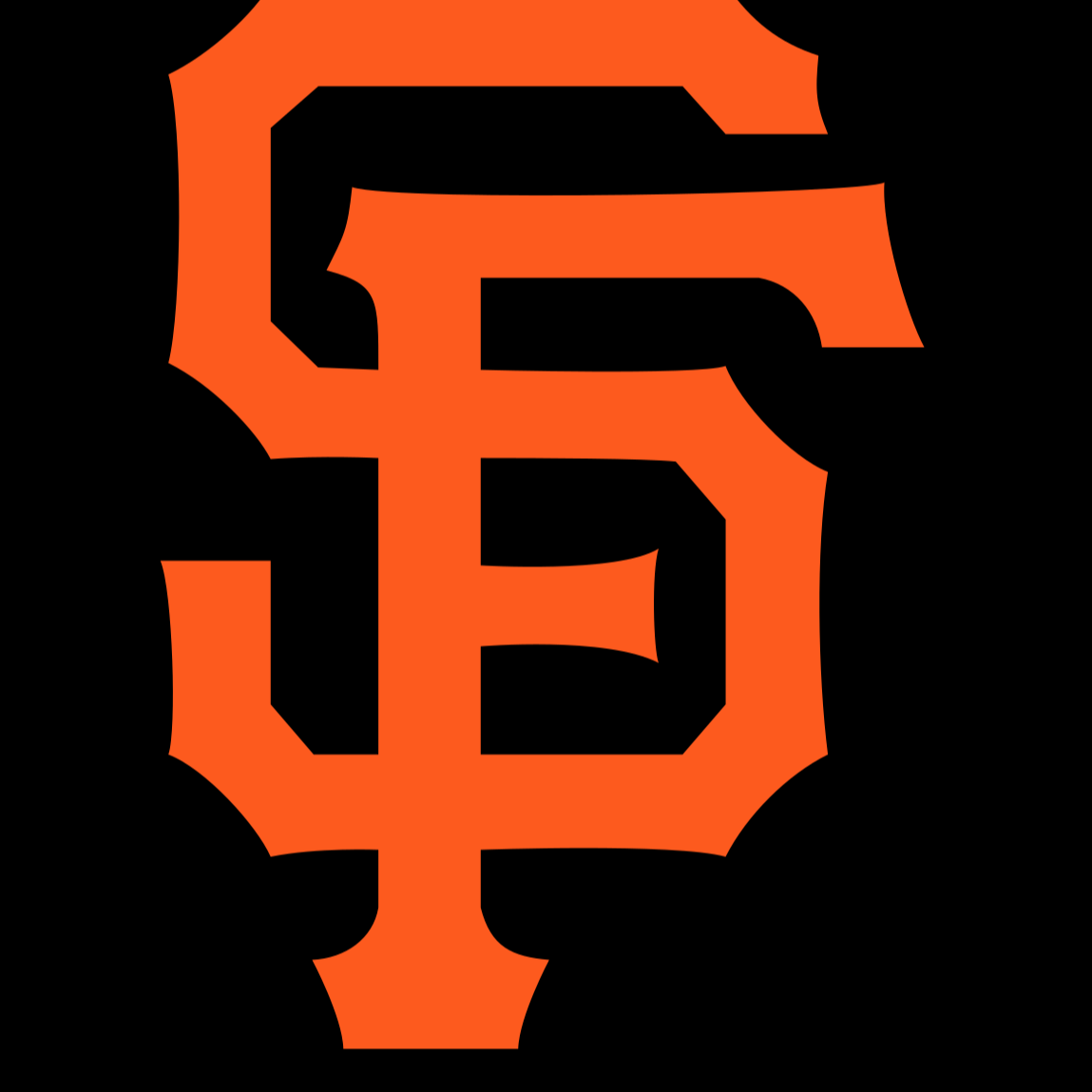###### #Python### Guessing Game

This is a Guessing game where you are supposed to use a while loop. In this game, While loops can be used to cycle through your code until you get the correct number.### Linear Regression with Multiple Variables### Linear Regression### Naive Bayes Algorithm

This is a program demonstrating the Naive Bayes Algorithm. It is used to see if it will be a good day to golf.### Hangman

I made a program for Hangman, where the user is given 5 wrong letters to try to guess the random word.### K Means Clustering

This program first assigns initial clusters to a set of data. It then takes the mean of each of these clusters and then uses them to make new clusters. If the new cluster is the same as the previous loop's cluster then the program ends.### Correlation Coefficient

A program that finds the correlation coefficient of two security companies(SPY and JPM) over a year and how each affect each other.Myles Brooks Jun 28

nice work### DNA to RNA to Amino Acids converter

Asks user to input length of DNA and makes a random DNA list and turns to RNA which turns to Amino Acids### Calculator

A calculator that can take an input of any number of digits and can add, subtract, multiply, and divideElijah Jun 28

myles doesn't mean that but nice work### KNN Algorithm (K Nearest Number)

This program asks the user for a specific point and an amount of neighbors to check. It then checks these nearest points for a color and the color of the most nearest points is the color of the user's point.### DNA to Amino Acid Converter

This program asks the user for a length of a DNA strand, it then generates the strand and converts it to RNA. After, it splits the RNA into Codons and then converts it into an Amino Acid Chain.### Converter for Decimal, Binary, Hexadecimal

This program converts decimal, binary, and hexadecimal...